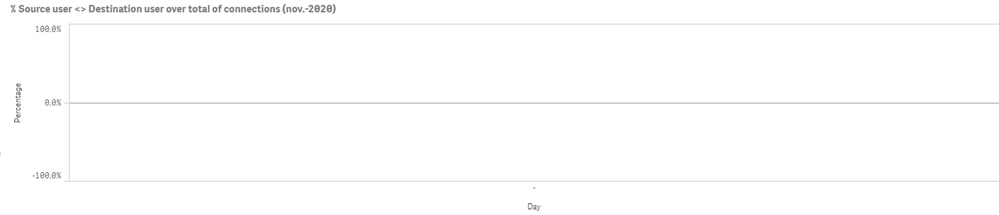# App Development

Announcements
Support Cases coming to Qlik Community Oct. 4! Start chats, open cases, explore resources: READ DETAILS
cancel
Showing results for
Did you mean:Partner

## Not showing current month

Hi all,

I want to show in a line chart the behavior of a KPI during the days of the current month. My expression is as follows:

=1+(

(count({\$<flag_user={"\$(='Sí')"},Month={"\$(=max(Month))"},Year={"\$(=max(Year))"}>}connect_time)
-
count({\$<Month={"\$(=max(Month))"},Year={"\$(=max(Year))"}>}connect_time)

)
/
count({\$<Month={"\$(=max(Month))"},Year={"\$(=max(Year))"}>}connect_time))

It works but only if a select the current month (november) in the filter pane, if not it shows up a  blank.

Without filters:November selected:So I want this last chart to show up without any selections.

Could anyone help me?

Labels (3)

• ### Set Analysis

1 Solution

Accepted SolutionsPartner

It seems that you have value for December for "Month" field (may be due to master calendar).

Just try changing your expression with something like this (calculating current month through today function)

=1+(

(count({\$<flag_user={"\$(='Sí')"},Month={"\$(=month(Today()))"},Year={"\$(=year(Today()))"}>}connect_time)
-
count({\$<Month={"\$(=month(Today()))"},Year={"\$(=year(Today()))"}>}connect_time)

)
/
count({\$<Month={"\$(=month(Today()))"},Year={"\$(=year(Today()))"}>}connect_time))

Hope it helps

Regards

2 RepliesPartner

It seems that you have value for December for "Month" field (may be due to master calendar).

Just try changing your expression with something like this (calculating current month through today function)

=1+(

(count({\$<flag_user={"\$(='Sí')"},Month={"\$(=month(Today()))"},Year={"\$(=year(Today()))"}>}connect_time)
-
count({\$<Month={"\$(=month(Today()))"},Year={"\$(=year(Today()))"}>}connect_time)

)
/
count({\$<Month={"\$(=month(Today()))"},Year={"\$(=year(Today()))"}>}connect_time))

Hope it helps

RegardsPartner
Author

Great Forte! It works!

Good workaround.

Thank you for your help. Regards.Tags
Community Browser